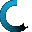Retrieving data ...
Please do not click anywhere else.

If you see no response after 2-3 minutes, then click on the [X] button in the upper right corner to close the window and retry the operation at a later time.
Interactive Multimedia Learning & Teaching Management System Suite## Course Contents

• 1. Counting and Identifying Numbers
•  1.1 Identifying and Counting Numbers to 100
•  1.2 Identifying and Counting Numbers past 100
•  1.3 Skip Counting by Two (up to 100)
•  1.4 Skip Counting by Five (up to 100)
•  1.5 Skip Counting by Ten (up to 100)
•  1.6 Skip Counting by Twenty-five (up to 1000)
•  1.7 Reverse Counting (starting at 100)
•  1.8 Reverse Skip Counting by Two (starting 100)
•  1.9 Reverse Skip Counting by Five (starting 100)
•  1.10 Reverse Skip Counting by Ten (starting 100)
• 2. Place Value, Writing Numbers and Number Combinations
•  2.1 Introduction to Place Values
•  2.2 Place Values for 3-digit Numbers
•  2.3 Writing Numbers Using Words (up to 100)
•  2.4 Write Numbers that are Given in Words (up to 100)
•  2.5 Number Combinations (up to 3 digits)
• 3. Naming, Comparing and Arranging Numbers
•  3.1 Name the Number
•  3.2 Comparing 2-Digit Numbers
•  3.3 Comparing 3-Digit Numbers
•  3.4 Arranging Numbers in Ascending Order
•  3.5 Arranging Numbers in Descending Order
•  3.6 Number Types
•  3.7 Even and Odd Numbers
•  4.5 Addition without Carrying Numbers (Optional)
•  4.6 Addition with Carrying Numbers
•  4.7 Adding a 2-digit Number and a 1-digit Number
•  4.8 Adding a 2-digit Number and a 2-digit Number
•  4.9 Adding a 3-digit Number and a 2-digit Number
•  4.10 Adding a 3-digit Number and a 3-digit Number
•  4.11 Adding 3 Number Sets
• 5. Subtraction
•  5.1 Introduction to Subtraction
•  5.2 Subtraction in Relation to Addition
•  5.3 Subtraction Using Objects
•  5.4 Subtraction Using a Subtraction Table
•  5.5 Subtraction Using Regrouping
•  5.6 Subtraction without Borrowing Numbers (Optional)
•  5.7 Subtraction with Borrowing Numbers
•  5.8 Subtracting a 1-digit Number from a 2-digit Number
•  5.9 Subtracting a 1-digit Number from a 3-digit Number
•  5.10 Subtracting a 2-digit Number from a 2-digit Number
•  5.11 Subtracting a 2-digit Number from a 3-digit Number
•  5.12 Subtracting a 3-digit Number from a 3-digit Number
• 6. Multiplication
•  6.1 Introduction to Multiplication
•  6.2 Multiplication in Relation to Addition
•  6.3 Multiplication Using Objects
•  6.4 Multiplication Using a Multiplication Table
•  6.5 Multiplication Tricks
•  6.6 Multiplying a 2-digit Number by a 1-digit Number
• 7. Division
•  7.1 Introduction to Division
•  7.2 Division in Relation to Subtraction
•  7.3 Division in Relation to Multiplication
•  7.4 Division Using Objects
•  7.5 Division with a Remainder
•  7.6 Division with 0 and 1
• 8. Rounding and Estimating
•  8.1 Rounding to the Nearest 10 using a Number Line
•  8.2 Rounding to the Nearest 100 using a Number Line
•  8.3 Rounding without using a Number Line
•  8.5 Estimating with Subtraction
• 9. Fractions
•  9.1 Introduction to Fractions
•  9.2 Identifying Fractions
•  9.3 Writing Fractions
•  9.4 Equivalent Fractions
•  9.5 Writing Equivalent Fractions
•  9.6 Comparing Fractions
•  9.8 Subtracting Fractions
• 10. Tables, Charts and Graphs
•  10.1 Introduction to Tables, Charts and Graphs
•  10.2 Creating Tally Charts
•  10.3 Interpreting Tally Charts
•  10.4 Creating Picture Graphs
•  10.5 Interpreting Picture Graphs
•  10.6 Creating Bar Graphs
•  10.7 Interpreting Bar Graphs
• 11. Algebra
•  11.1 Patterns
•  11.2 Arithmetic Number Patterns
•  11.3 Geometric Number Patterns
•  11.4 Find the Missing Number
•  11.5 Properties of Addition and Multiplication
•  11.6 Expressions and Equations
•  11.7 Writing Expressions and Equations
•  11.8 Completing Equations
• 12. Geometry
•  12.1 Basic 2-dimensional Geometric Shapes
•  12.2 Identifying 2-dimensional Shapes
•  12.3 Polygons
•  12.4 Basic 3-dimensional Geometric Shapes
•  12.5 Identifying 3-dimensional Shapes
•  12.6 Working with Shapes
•  12.7 Identifying Congruent 2-dimensional Shapes
•  12.8 Lines of Symmetry
•  12.9 Completing the Shape
• 13. Time
•  13.1 Days, Weeks, Months and Years
•  13.2 Days
•  13.3 Weeks
•  13.4 Months
•  13.5 Years
•  13.6 Converting Time: Days, Weeks, Months and Years
•  13.7 The Four Seasons
•  13.8 Seconds, Minutes and Hours
•  13.9 Digital and Analog Clocks
•  13.10 Telling Time to the Hour
•  13.11 Telling Time to the Half-hour
•  13.12 Telling Time to the Quarter-hour
•  13.13 Telling Time to 5 Minute Intervals
•  13.14 Converting Time: Seconds, Minutes and Hours
•  13.15 Duration of Time
• 14. Money
•  14.1 Pennies, Nickels, Dimes, Quarters
•  14.2 Converting Nickels, Dimes and Quarters to Pennies
•  14.3 Converting Pennies to Nickels, Dimes and Quarters
•  14.4 Counting Coins up to One Dollar
•  14.5 Counting Coins Past One Dollar
•  14.6 Dollars
•  14.7 Counting Dollars and Coins
•  14.8 Comparing Money
• 15. Measurements
•  15.1 Systems of Measurement
•  15.2 The U.S. System of Measurement
•  15.3 The Metric System of Measurement
•  15.4 Length
•  15.5 Perimeter and Area
•  15.6 Volume
•  15.7 Weight
•  15.8 Temperature